# AP Calculus AB : Corresponding characteristics of graphs of ƒ and ƒ'

## Example Questions

### Example Question #1 : Introduction To Derivatives

The speed of a car traveling on the highway is given by the following function of time: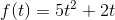Consider a second function: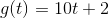It represents another way to write the car's speed.

It represents the total distance the car has traveled at time.

It has no relation to the previous function.

It represents the change in distance over a given time.

It represents the rate at which the speed of the car is changing.

It represents the rate at which the speed of the car is changing.

Explanation:

Notice that the function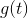is simply the derivative of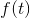with respect to time. To see this, simply use the power rule on each of the two terms.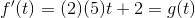Therefore,is the rate at which the car's speed changes, a quantity called acceleration.

### Example Question #2 : Derivative As A Function

Find the critical numbers of the function,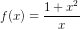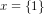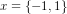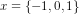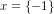Explanation:1) Recall the definition of a critical point:

The critical points of a function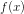are defined as points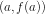, such thatis in the domain of, and at which the derivative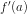is either zero or does not exist. The numberis called a critical number of.

2) Differentiate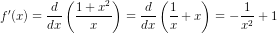3) Set to zero and solve for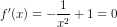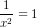The critical numbers are,We can also observe that the derivative does not exist at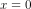, since the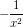term would be come infinite. However,is not a critical number because the original functionis not defined at. The original function is infinite at, and thereforeis a vertical asymptote ofas can be seen in its' graph,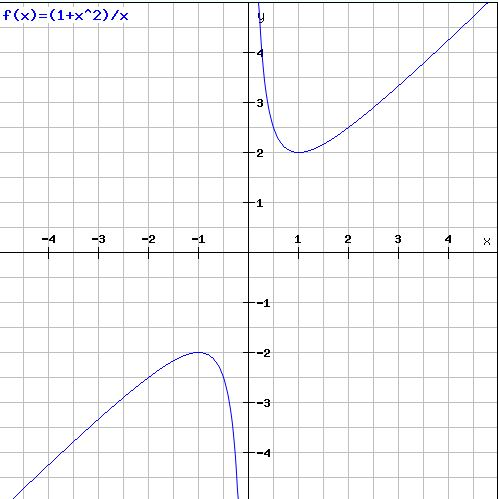Further Discussion

In this problem we were asked to obtain the critical numbers. If were were asked to find the critical points, we would simply evaluate the functionat the critical numbers to find the corresponding function values and then write them as a set of ordered pairs,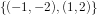### Example Question #3 : Derivative As A Function

The functionis a continuous, twice-differentiable functuon defined for all real numbers.

If the following are true:

•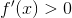•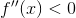Which function could be?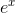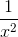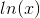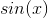Explanation:

To answer this problem we must first interpret our given conditions:

•Implies the function is strictly increasing.
•Implies the function is strictly concave down.

We note the only function given which fufills both of these conditions is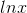.

### Example Question #171 : Derivatives

A jogger leaves Cityat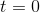.  His subsequent position, in feet, is given by the function: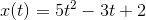,

whereis the time in minutes.

Find the acceleration of the jogger at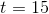minutes.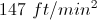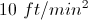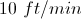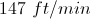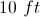Explanation:

The accelaration is given by the second derivative of the position function: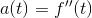For the given position function: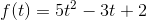,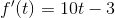,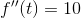.

Therefore, the acceleration atminutes is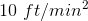.  Again, note the units must be in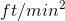.